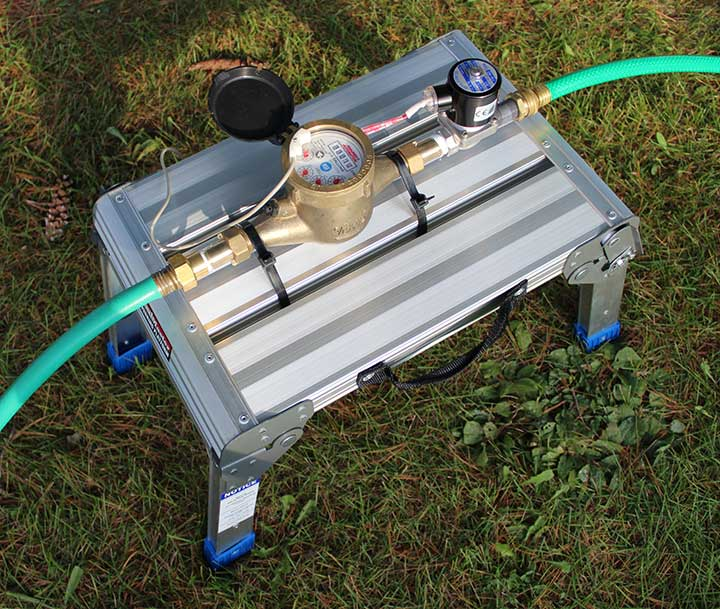855-871-6091
We Are Water Meter Experts

# Pool Filling System

Select a Batching System

The ABC-2020 batch controller is a perfect solution for pond and pool filling. The ABC-10 program allows an operator to set the amount in increments of 10 gallons. First calculate or measure the amount of gallons it takes to raise the water level 1 inch. Then multiply that by the number of inches you want to the current level to rise. Set the ABC to dispense that amount and press the Start button.### Calculating How Much Water to Add

We recommend that pool owners calculate how many gallons = 1 inch in water level rise. This can be done in 2 ways:

Method 1: Use some basic math

1. Calculate the area in square feet

Rectangular Pools:
Width ft. x Length ft. = Area ft2

Circular Pools:
Radius (in feet) Squared x Pi (3.14) = Area ft2

Oval Pools:
1/2 width ft. x 1/2 Width ft. x Pi (3.14) = Rounded Sections Area ft2
Width ft. x (Length ft. - Width ft.) = Rectangular Area ft2
Rounded Sections Area ft2 + Rectangular Area ft2 = Total Area ft2

Kidney or Other Complex Pool footprints:
Use Method 2

2. Calculate the Volume of a 1 foot rise in Cubic Feet

Multiply the area by 1 ft. to get cubic feet of a one foot rise.
Area ft2 x 1 ft = Volume ft3

3. Calculate the Volume of a 1 inch rise in Cubic Feet

Divide the Volume ft3 by 12 to get the cubic feet needed for a 1 inch rise.

4. Convert the Cubic Feet into Gallons

Multiply the cubic feet by 7.48052 to convert it to Gallons.

5. Set your ABC batcher to that amount x the number of inches that you want the water to rise and hit start.

Method 2: Dispense and Measure the Rise of a Know Amount

1. Mark or otherwise take note of the current water level.

2. Set the batch controller for a high volume (100 gallons or more but use a nice round number) and run a batch.

3. Note the new water level and measure the change in inches. If the level rise is less than an inch, run another batch. Keep running batches until a  noticeable rise has been achieved and measured. Be sure to keep track of the total gallons added.

4. calculate the Volume of a 1 inch rise.

Total Gallons Dispensed / inches of rise = Gallons per inch

5. Now record this value, so the next time you need to add water, you can simply multiply that number of gallons by the number of inches that you want.

Select a Batching System